EdgeForm

The next cell displays the usage message for EdgeForm.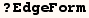The usage message for EdgeForm indicates  EdgeForm is a "three-dimensional graphics directive" this indicates it can be used inside Graphics3D and with functions that produce Graphics3D expressions.  ParametricPlot3D produces Graphics3D, and it's usage message indicates that ParametricPlot3D[{fx,fy,fz,s},{x,xmin,xmax},{ymin,ymax}] produces a plot shaded according to the color specification (s).  Here (s) can be a single three-dimensional graphics directive or a list of three-dimensional graphics directives.

Consider the next cell where the default EdgeForm around polygons is used.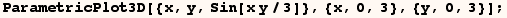The next cell makes the same graphic except no edges are drawn around the polygons.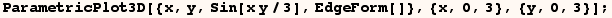The next cell plots the same function using a gray edges around the polygons.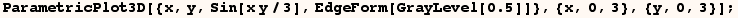The next cell uses colored edges where the color varies as a function of x and y.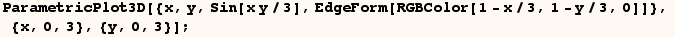The next cell uses thick colored edges where the color varies as a function of x and y.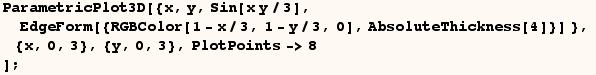An example is given below where EdgeForm is used with Grapgics3D.  Here EdgeForm[] indicates no edges should be drawn around the polygons.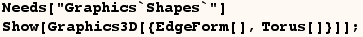In the next cell Gray edges around the polygons are used.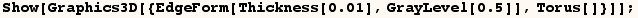Keep in mind that DensityPlot, ListDensityPlot, ListPlot3D, Plot and Plot3D don't produce Graphics3D expressions.  Instead one should use the options Mesh and MeshStyle to get similar results with DensityPlot, ListDensityPlot, ListPlot3D, Plot and Plot3D.

Created by Mathematica  (May 16, 2004)

Back to Ted’s Tricks index page